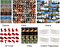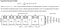# Deep Energy-Based Generative Models

Energy-based generative modeling and learning have been successful in representing different types of data formats, such as images [1, 2, 3], videos [4, 5], 3D voxels [6, 7], and trajectories . Their successes are attributed to the invention of the energy-based Generative ConvNet  framework initially proposed by Song-Chun Zhu’s group from UCLA in 2016. The Generative ConvNet parameterizes its energy function by a bottom-up deep neural network and bridges the gap between deep learning and energy-based learning.Figure 1: The synthesized results generated by the energy-based Generative ConvNet family

# Generative PointNet

Recently, UCLA and Baidu propose a novel energy-based representational model for unordered point clouds by designing an input-permutation-invariant energy function for the generative ConvNet. They called the model, the Generative PointNet (GPointNet) .

The GPointNet is the first energy-based model for point cloud representation. It tries to directly learn a probability distribution of point clouds. It belongs to a descriptive model because this model tries to capture sufficient statistics from the data so that it can fully describe the data. Compared to previous GAN-based models, GPointNet does not rely on any extra assisting network and any human-designed point set distance metrics.

This work takes a further step for the energy-based model because it generalizes the existing energy-based framework that is suitable for regular data, such as images, videos, to unordered point sets. Meanwhile, it is the first energy-based model to perform point cloud reconstruction and interpolation. This can be achieved by using a short-run MCMC sampling to train the model, in which the Gaussian-initialized short-run MCMC can be treated as a multilayer flow-based generator where the initialization is the continuous latent variables.

You can access the code and pre-trained model on Github.

# History of 3D Deep Learning on Point Clouds

Point cloud uses a set of unordered point coordinates to represent a 3D object. It is a standard 3D acquisition format used by devices like Lidar on autonomous vehicles, Kinect for Xbox and face identification sensor on phones. The special part of the point cloud data compared to other data formats is that the point cloud is an unordered set, which means it has a permutation-invariant property. That is, changing the input order will not change the 3D structure of the point cloud.

In 2016, Qi proposed the PointNet , a permutation-invariant discriminative deep neural network. This opens a door for 3D deep learning on point clouds. The PointNet model has inspired the advance of discriminative models for 3D point cloud classification and segmentation.

The GPointNet is the generative modeling of the PointNet by turning the discriminative model into a generative model. According to the Bayes rule, they are equivalent to each other.

# Models and Learning Algorithm for Generative PointNet

## Model

A major challenge in modeling 3D point clouds is that unlike images, videos and volumetric shapes, point clouds are not regular structures but unordered point sets, which makes extending the existing energy-based paradigms intended for structured data not straightforward.

See Figure 2, the Generative PointNet (GPointNet) is an energy-based model on unordered point clouds, where the energy function is parameterized by a well-designed input-permutation-invariant bottom-up network f. The normalization constant Z is typically intractable. p0(X) is a known reference distribution such as Gaussian white noise distribution. The Generative PointNet is also called the exponential tilting of a Gaussian reference distribution.

The input-permutation-invariant bottom-up network f first takes all unordered points as input, encodes each point into features by multi-layer perceptron (MLP), and then aggregates all point features to a global feature by average pooling, and eventually outputs a scalar by another MLP.Figure 2: Model and network architecture of energy-based Generative PointNet (GPointNet)

## Learning

The model can be learned by maximum likelihood estimation (MLE), which tries to find model parameters that can assign higher scores (i.e., lower energies) to those observed training point clouds and assign lower scores (i.e., higher energies) to those unobserved ones. The gradient of the log-likelihood is given by

The second expectation term is intractable due to the intractable normalizing constant, therefore the learning algorithm relies on Markov chain Monte Carlo (MCMC) sampling, such as Langevin dynamics, to approximate the expectation by the sample average.

See Figure 3. The learning algorithm follows “analysis by synthesis”, which iterates the sampling step and the learning step. To be specific, in the sampling step, Langevin dynamics is used to draw samples from the distribution. With the synthesized examples and the observed examples, the model parameters are updated by a gradient-based optimizer in the learning step.

There are different implementations of the Langevin dynamics sampling step: (i) Persistent chain: runs a finite-step MCMC from the synthesized examples generated from the previous epoch. (ii) Contrastive divergence: runs a finite-step MCMC from the observed examples. (iii) Non-persistent short-run MCMC: runs a finite-step MCMC from Gaussian white noise. The GPointNet model adopts short-run MCMC to train the model, such that it can unify the generation, reconstruction, and interpolation into a single framework.

# Understanding Short-Run MCMC as a Latent Variable Model

The GPointNet uses a short-run MCMC to train the model. The short-run MCMC performs finite steps (e.g., 64 steps) of Langevin steps which is actually not convergent to the target distribution. That is, the sampled X is highly dependent on the initialization Z of the sampling process. Thus, this sampling process can be considered a generator where the continuous latent vector is Z. This generator can act the same as the ordinary generator.

Each step in the sampling process can be regarded as one layer with noise injected into the residual network. The whole short-run MCMC generator then becomes a 64-layer residual network with noise injected into each layer. Let M be the transition kernel of K=64 steps of MCMC toward the model. For a fixed initial probability p0, the resulting marginal distribution of sample X after running K steps of MCMC starting from p0 is denoted by

Training θ with short-run MCMC is no longer a maximum likelihood estimator (MLE) but a moment matching estimator (MME) that solves the following estimating equation:

which is a perturbation of the maximum likelihood estimating equation.

# Experimental Results

## Generation

The generation process for an energy-based model is the Langevin sampling on the trained probability distribution. Figure 4 shows the result generated by the learned model trained on the ModelNet10 dataset.

## Reconstruction

It is worth mentioning that the GPoinNet is trained by the short-run MCMC, which can be treated as a latent variable model or flow-based generator so that the model can be useful for point cloud reconstruction. GPointNet is the first energy-based model to perform point cloud reconstruction. Figure 5 shows the reconstruction results by the learned short-run MCMC.

## Interpolation

Since the short-run MCMC is a latent variable model, interpolation can be performed in the latent space to demonstrate that the learned latent space is meaningful. Figure 6 are some examples. The transition in each row displays the sequence of reconstruction with the linear interpolated latent variable Z.

## Classification

The learned point encoder in the score function f can be useful for point cloud feature extraction, and the features can be applied to supervised learning for point cloud classification. The procedure is given by: (1) Train a single GPointNet on the training examples from all categories. (2) Replace the average pooling layer with the max-pooling layer in the learned score function, and use the output of the max-pooling as point cloud features. (3) Train an SVM classifier from the labeled data based on the extracted features for classification.

See Figure 7 for an illustration. The table shows the classification accuracy using the one-versus-all rule. The tables show the robustness tests. Considering three types of data corruptions: Type 1 is missing points, where points are randomly deleted from each point cloud. Type 2 is added points, where extra points uniformly distributed are added into each point cloud. Type 3 is point perturbation, where each point of each point cloud is perturbed by adding Gaussian noise.

Classification accuracy of the classifier on the corrupted version of point cloud data is shown in the tables. The performance decreases as the corruption level increases. In the case of missing point corruption, even though 94% of points are deleted in each example, the classifier can still perform with an accuracy of 90.20%. In the extreme case where only 20 points (1%) are kept in each point cloud, the accuracy becomes 53.19%.

# Discussion

Modeling: GPointNet is a novel energy-based model that explicitly represents the probability distribution of an unordered point set, e.g., a 3D point cloud, by designing an input-permutation invariant bottom-up network as the energy function. This is the first generative model that provides an explicit density function for point cloud data. It will shed new light not only on the area of 3D deep learning but also on the study of unordered set modeling.

Learning: An unconventional short-run MCMC is used to learn the model and the MCMC is treated as a flow-based generator model, such that it can be used for point cloud reconstruction and generation simultaneously. Usually an energy-based model is unable to reconstruct data. This is the first energy-based framework that can perform point cloud reconstruction and interpolation.

Uniqueness: Compared with existing point cloud generative models, the GPointNet model has the following unique properties: (1) It does not rely on an extra assisting network for training. (2) It can be derived from the discriminative PointNet. This is why the model is called generative PoinNet. (3) It unifies synthesis and reconstruction in a single framework. (4) It unifies an explicit density (i.e., EBM) and an implicit density (i.e., short-run MCMC as a latent variable model) of the point cloud in a single framework.

Performance: Competitive performances are contained with much fewer parameters compared with the state-of-art point cloud generative models, such as GAN-based and VAE-based approaches, in the tasks of synthesis, reconstruction and classification.

# Reading Source: Tutorial on Energy-Based Generative Models

People who are interested in energy-based generative models can watch the CVPR 2021 tutorial on theory and application of energy-based generative models at: https://energy-based-models.github.io/ for current advances of the EBMs.

# Reference

 A Theory of Generative ConvNet. Jianwen Xie *, Yang Lu *, Song-Chun Zhu, Ying Nian Wu (* equal contributions) (ICML 2016)

 Learning Generative ConvNets via Multigrid Modeling and Sampling. Ruiqi Gao*, Yang Lu*, Junpei Zhou, Song-Chun Zhu, and Ying Nian Wu (* equal contributions)(CVPR 2018).

 On Learning Non-Convergent Non-Persistent Short-Run MCMC Toward Energy-Based Model. E Nijkamp, M Hill, Song-Chun Zhu, and Ying Nian Wu (NeurIPS 2019)

 Synthesizing Dynamic Pattern by Spatial-Temporal Generative ConvNet. Jianwen Xie, Song-Chun Zhu, Ying Nian Wu (CVPR 2017)

 Learning Energy-based Spatial-Temporal Generative ConvNet for Dynamic Patterns. Jianwen Xie, Song-Chun Zhu, Ying Nian Wu (TPAMI 2020)

 Learning Descriptor Networks for 3D Shape Synthesis and Analysis. Jianwen Xie *, Zilong Zheng *, Ruiqi Gao, Wenguan Wang, Song-Chun Zhu, Ying Nian Wu (* equal contributions)(CVPR 2018)

 Generative VoxelNet: Learning Energy-Based Models for 3D Shape Synthesis and Analysis. Jianwen Xie *, Zilong Zheng *, Ruiqi Gao, Wenguan Wang, Song-Chun Zhu, Ying Nian Wu (* equal contributions) (TPAMI 2020)

 Energe-based Continous Inverse Optimal Control Yifei Xu, Jianwen Xie, Tianyang Zhao, Chris Baker, Yibiao Zhao, Ying Nian Wu (NeurIPS workshop on Machine Learning for Autonomous Driving, 2020)

 Generative PointNet: Energy-Based Learning on Unordered Point Sets for 3D Generation, Reconstruction and Classification. Jianwen Xie *, Yifei Xu *, Zilong Zheng, Song-Chun Zhu, Ying Nian Wu (* equal contributions)(CVPR 2021)

 PointNet: Deep learning on point sets for 3D classification and segmentation. Charles R Qi, Hao Su, Kaichun Mo, and Leonidas J Guibas. （CVPR 2016)